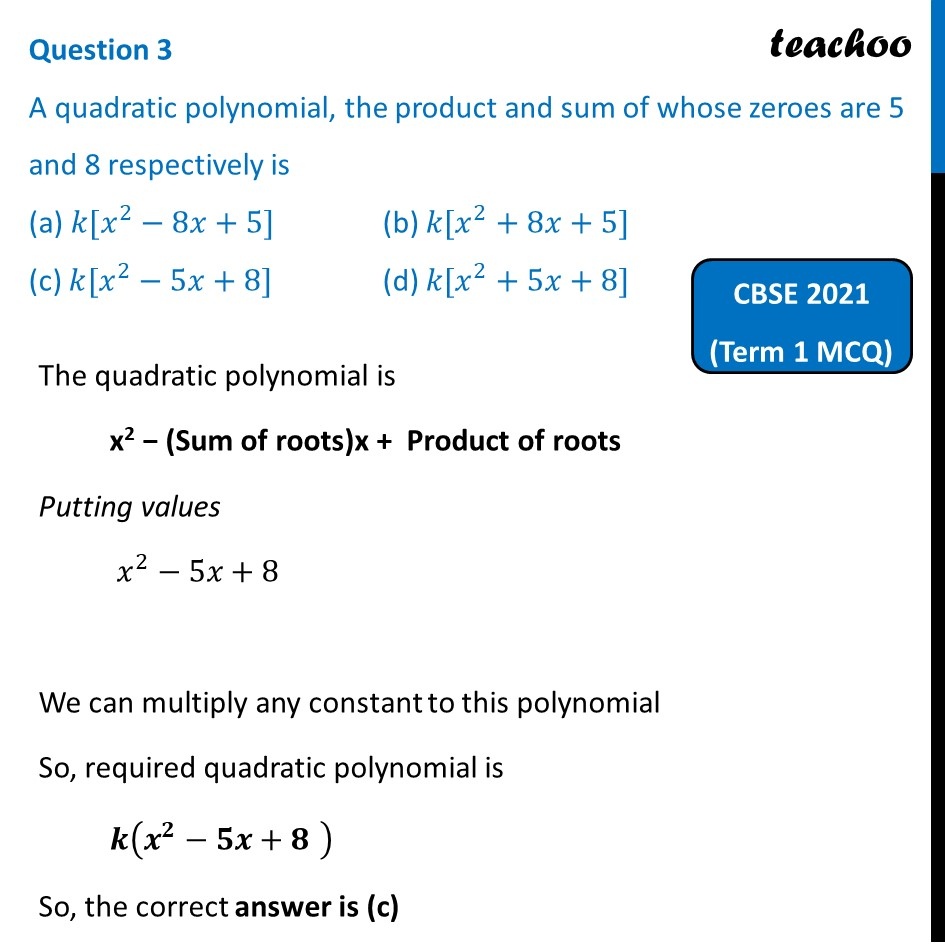Past Year MCQ (Maths Standard)

Chapter 2 Class 10 Polynomials
Serial order wise

## (d) k[x 2 + 5x + 8]

This question is similar from Question 2 - CBSE Class 10 - Sample Paper for 2021 Boards - Maths BasicLearn in your speed, with individual attention - Teachoo Maths 1-on-1 Class

### Transcript

Question 3 A quadratic polynomial, the product and sum of whose zeroes are 5 and 8 respectively is (a) 𝑘[𝑥^2−8𝑥+5] (b) 𝑘[𝑥^2+8𝑥+5] (c) 𝑘[𝑥^2−5𝑥+8] (d) 𝑘[𝑥^2+5𝑥+8] The quadratic polynomial is x2 − (Sum of roots)x + Product of roots Putting values 𝑥^2−5𝑥+8 We can multiply any constant to this polynomial So, required quadratic polynomial is 𝒌(𝒙^𝟐−𝟓𝒙+𝟖" " ) So, the correct answer is (c)